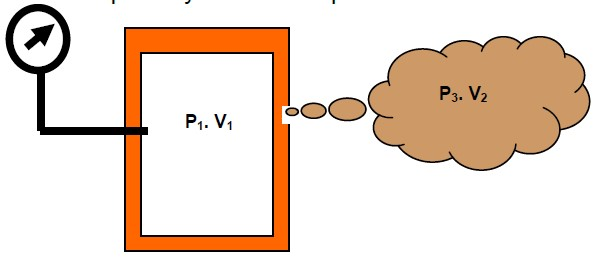# The Relationship Between Pressure Decay & Leak Rate

By default, pressure decay panels measure change in pressure to indicate the presence of a leak in a pressurized test piece e.g., mbar, Pascal, mbar/sec or Pascal/sec. However, leak specifications often quote a volumetric specification such as cc/sec or cc/min.

It is possible to convert a pressure decay test panel to read in volumetric units once the total test volume of the part under test is known. This information becomes available once a test system is built and must also take into consideration the total test volume which included the test part, the test connection pipe and the internal volume of the test unit.

The relationship between pressure drop, leak rate and volume is derived from the basic gas law and is as follows:

Pressure Drop (mbar / sec) = Leak Rate ÷ Total Test Volume

Note: The temperature term in the basic gas law is considered to be constant for purposes of leak detection systems and therefore does not appear in the formula above. The justification for this lies in the use of stabilization time which is designed to delay the test phase to a point where adiabatic temperature effects have been dissipated.

### Worked Example

This example is an illustration that applies the theory to your test circumstance, the test criteria is as follows:

• Test pressure 200 psig
• Reject level is 0.17 psi in 10 seconds (this is equal to 0.017psi/sec.)
• The tester internal volume is 7 ccs, we should also add 0.5 cc for fittings and test part combined, making a total volume of 7.5 ccs.

To determine the leak rate the above equation is transposed to make the leak rate the subject.

Leak Rate = Pressure Drop x Volume ÷ Atmospheric Pressure

Therefore, the leak rate in cc/sec will become:

= 0.017 x 7.5 = 0.00867 cc/sec = 0.52
cc/min.
14.7

The test volume is an estimate based on the design parameters of the Sprint LC, there will be slight variation from unit to unit, and therefore it would be prudent to round the calculated value down to 0.5

cc/min.

To specify a leak master the leak rate and test pressure must be quoted. In this case the leak master should be quoted as 0.5 cc/min @ 200 psig.

### Development of the Pressure: Drop ~ Leak Rate Relationship

The formula shown in the above section can be proven from the basic gas laws as follows:

Consider a sealed vessel where the internal pressure is not equal to the ambient or external pressure.

When pressurized the vessel has a quantity of air at time t0 represented by its volume multiplied by the

internal pressure V1 x P1.If the pressure supply is removed and the volume sealed and there was a small leak present the internal pressure would fall such that after time t1 the resultant amount of air in the vessel would equate to volume V1 and Pressure P2. Note, the volume is considered to be constant under the test conditions. The amount of gas leaked would be at atmospheric pressure P3 and occupy a volume V2. The initial quantity of air in the vessel at time to (V1 x P1) will be represented by the same quantity of air at time t1 in two parts (V1 x P2 and V2 x P3), which is represented by the following equation:

V1 x P1 = (V1 x P2) + (V2 x P3)

By transposition the internal volume can be brought to one side as follows:

(V1 x P1) – (V1 x P2) = (V2 x P3)

Then rationalizing the LH side V1(P1-P2) = V2 x P3 and isolating the pressure drop term P1-P2 P1-P2 = V2 x P3 V1.

So far, we have not included the time difference between the time t0 and t1. Adding the time into the relationship we get P1-P2 = V2 x 1 x P3 (where t would be normalized to a unitary value, typically 1 sec)

t t V1. Therefore, to rationalize P1-P2/ t becomes pressure drop rate V2/t becomes a volumetric flow rate V1 Is the total test volume P3 Is atmospheric pressure.

The relationship can then be expressed as:

Pressure Drop / sec = Leak Rate / sec x Total Test Volume

We can now choose the units we wish to work in either in imperial, MKS or SI and calculate the pressure drop from the leak rate and volume, or by transposition calculate the leak rate that created the pressure drop; providing we know the test volume.

### Transfer Standard

A leak test specification that specifies a leak rate; relates a leak size to a test pressure and therefore is a standard measurement that can be used to challenge any leak test system. By comparison a leak test specification that specifies a pressure drop is masked from the leak site by variations in test volume. The larger the test volume to slower the pressure will drop for a given leak.